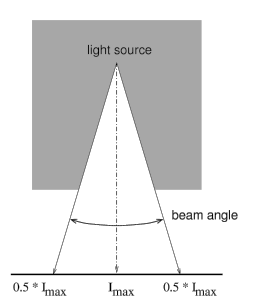# Beam Angle CalculatorThis convenient calculator will determine the beam angle, the beam spot size, or the distance of a beam.

How to use it:

Just enter two known values, and click the calculate button to calculate the unknown value you are looking for.  For instance, if you wish to know the coverage area  (spot size) of a 10° beam mounted 25 feet off of the deck (throw distance), just enter these two values, and click the Calculate Spot Size to find the diameter of the spot.  In this case the result is 4.37 feet.

Any value can be determined as long as you know at least two of the variables.  The calculator assumes a symmetrical beam (round beam). You may use any unit (feet, meters, etc.) for the distance and spot size, but the beam angle must be in degrees.

 Throw Distance Units Beam Angle Degrees Spot Size Units

How is a beam measured?

While the above calculator uses trigonometry to calculate the theoretical angle, in the world of lighting we measure actual beam angles using the Full Width Half Maximum (FWHM) Angle method.  All this means is that because it is not practical to measure light on both sides of a beam out indefinitely, we only measure the light to the angle where the intensity falls below 50% of the initial intensity in the center of the beam.  In other words, we find the center of the beam and take an intensity measurement. We then move to the side of the beam until the intensity is 50% of the initial reading.  That angle is the half-maximum-angle. We then multiply that half-angle by two to get the Full Width Half Maximum Angle (FWHM).